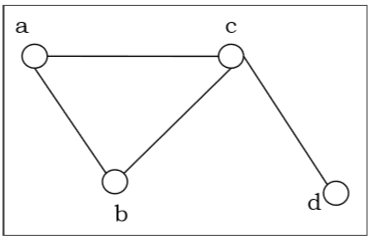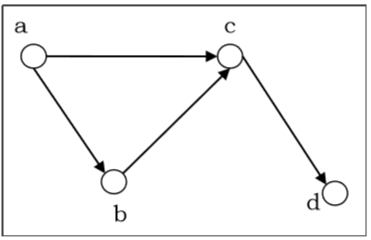# Adjacency Matrices and their properties

Adjacency Matrix is used to represent a graph. We can represent directed as well as undirected graphs using adjacency matrices. Following are the key properties of an Adjacency matrix.

## Properties

• An Adjacency Matrix A[V][V] is a 2D array of size V × V where V is the number of vertices in a undirected graph.

• If there is an edge between Vx to Vy then the value of A[Vx][Vy] = 1 and A[Vy][Vx]=1, otherwise the value will be zero.

• For a directed graph, if there is an edge between Vx to Vy, then the value of A[Vx][Vy]=1, otherwise the value will be zero.

Adjacency Matrix of an Undirected Graph

Let us consider the following undirected graph and construct the adjacency matrix −Adjacency matrix of the above undirected graph will be −

 a b c d a 0 1 1 0 b 1 0 1 0 c 1 1 0 1 d 0 0 1 0

Adjacency Matrix of a Directed Graph

Let us consider the following directed graph and construct its adjacency matrix −Adjacency matrix of the above directed graph will be −

 a b c d a 0 1 1 0 b 0 0 1 0 c 0 0 0 1 d 0 0 0 0